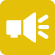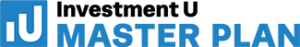## 1-Minute Survey

What Type of Investor Are You? – Take This 1-Min Survey to Find Out

Financial Literacy

# What are the Types of Interest?

No matter which side of a financial transaction you’re on, it’s important to understand the concept of interest. It’s the charged fee for borrowing money—often a percentage of the amount borrowed. For lenders, it’s what they can expect to make from the transaction. For borrowers, it’s the cost of that transaction.

While the concept of interest remains the same, it takes different forms. The type of interest you’re dealing with depends on the nature of the investment, the amount, the term and who’s involved in the transaction. In total, there are seven chief types of interest.## 1. Simple Interest

This is the rate charged by banks for borrowing money. It’s calculated by multiplying the daily interest rate by the principal, then by the number of days between payments. Simple interest doesn’t compound. The equation looks like this:

A = P (1 + rt)

• A is the final amount
• P is the initial principal balance
• r is the annual interest rate
• t is the term

You’ll typically encounter simple interest for most personal loans, auto loans and other short-term loan products. While it’s easy to evaluate different loans and rate options by looking at simple interest, note that it doesn’t account for loan fees.

## 2. Compound Interest

Every investor should be familiar with compound interest. It is, after all, what’s responsible for wealth generated by investments. The longer the term and the more compounding periods, the more money paid in interest. The equation for compound interest looks like this:

A = P (1 + r/n)nt

• A is the final amount
• P is the initial principal balance
• r is the interest rate
• n is the number of times interest applied per time period
• t is the number of time periods elapsed

Compound interest takes into account the accrued value of interest payments with each new compounding event. To see exactly how powerful compound interest is, plug some figures into our compound interest calculator and see the returns for yourself!

## 3. Fixed Interest

Fixed interest doesn’t change. It’s a single interest rate tied to an original principal balance and represents the total amount the borrower must repay over the term—or how much the lender will gain. The formula looks like this:

A = P x r

• A is the final amount
• P is the initial principal balance
• r is the interest rate

Fixed interest tends to accompany some types of loans, but is most associated with bonds. Investors buy bonds with a clear understanding of how much they’ll earn on their investment based on the bond’s interest rate.

## 4. Variable Interest

Interest rates aren’t always static. Variable interest rates represent a rate that has the power to change monthly or annually, depending on the product and the rate environment. To calculate the total interest generated by a variable interest rate, you need to calculate the simple interest rate for each period, factoring in the new rate for each calculation. The cumulative interest is the sum of each period’s interest generated.

Variable interest crops up across different investment and loan products. Equity investments don’t have the same rate of return year-over-year, and Adjustable-Rate Mortgages (ARMs) change in relation to the prime rate. Variable rates can be great for borrowers in rate-friendly environments, but encumbering as rates rise.

## 5. Annual Percentage Rate (APR)

Most often associated with credit cards, APR represents total interest, expressed annually on the total cost of the loan. Credit companies formulate APR by taking the prime rate and building in a margin. The formula for APR looks like this:

APR = (((F+I/P)/n) x 365) x 100

• F represents fees
• I is the total interest paid over the life of the loan
• P is the principal balance
• n is number of days in the loan term

APR is a useful tool for comparing loans; however, it can also be a deceiving figure. It’s vital to distinguish between APR and Annual Percentage Yield (APY), which takes into account compounding. As a result, APR isn’t an accurate reflection of the total cost of borrowing.

## 6. Prime Rate Interest

The prime rate is the best possible interest rate banks offer for loan products. It’s reserved for only the most creditworthy borrowers, and banks use it as the benchmark for marking up the interest rates on their financial products.

Where does the prime rate come from? It’s set by central banks and the federal reserve. The lower this rate is, the lower consumer interest rates drop and the easier it is to borrow money. Likewise, the higher the prime rate, the higher secondary and tertiary rates are, making it more difficult to borrow.

## 7. Discount Interest Rate

The discount interest rate is a type of interest that isn’t consumer-facing. Instead, this is the rate at which central banks and the federal reserve use when lending money to other financial institutions. This rate covers short-term loans primarily used to help correct liquidity and cover funding shortages. The rate is extremely low as not to hamper the borrowing institution.

## Get Familiar With Interest Rates

If you’re borrowing or lending money in any capacity, interest is an all-important factor in how that transaction will play out. Make sure you know the interest rate and the types you’re working with. More importantly, make sure you have the tools to extrapolate it, so you know how much money you’re spending or making on a transaction. Doing the math on a loan or an investment product with a given interest rate will clue you in to exactly what to expect over the term of the transaction. And this can help you find your path to financial freedom. To learn more, sign up for the Liberty Through Wealth e-letter below.

###### Articles by Leanna Kelly